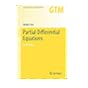Normal view

# Partial Differential Equations [electronic resource] / by Jürgen Jost.

Material type:TextSeries: Graduate Texts in Mathematics: 214Publisher: New York, NY : Springer New York : Imprint: Springer, 2013Edition: 3rd ed. 2013Description: XIV, 410 p. online resourceContent type: text Media type: computer Carrier type: online resourceISBN: 9781461448099Additional physical formats: Printed edition:: No titleDDC classification: 515.353 LOC classification: QA370-380Online resources: Click here to access online
Contents:
Preface -- Introduction: What are Partial Differential Equations? -- 1 The Laplace equation as the Prototype of an Elliptic Partial Differential Equation of Second Order -- 2 The Maximum Principle -- 3 Existence Techniques I: Methods Based on the Maximum Principle -- 4 Existence Techniques II: Parabolic Methods. The Heat Equation -- 5 Reaction-Diffusion Equations and Systems -- 6 Hyperbolic Equations -- 7 The Heat Equation, Semigroups, and Brownian Motion.- 8 Relationships between Different Partial Differential Equations -- 9 The Dirichlet Principle. Variational Methods for the Solutions of PDEs (Existence Techniques III) -- 10 Sobolev Spaces and L^2 Regularity theory -- 11 Strong solutions -- 12 The Regularity Theory of Schauder and the Continuity Method (Existence Techniques IV) -- 13The Moser Iteration Method and the Regularity Theorem of de Giorgi and Nash -- Appendix: Banach and Hilbert spaces. The L^p-Spaces -- References -- Index of Notation -- Index.
Summary: This book offers an ideal graduate-level introduction to the theory of partial differential equations.  The first part of the book describes the basic mathematical problems and structures associated with elliptic, parabolic, and hyperbolic partial differential equations, and explores the connections between these fundamental types. Aspects of  Brownian motion or pattern formation processes are also presented. The second part focuses on existence schemes and develops estimates for solutions of elliptic equations, such as Sobolev space theory, weak and strong solutions, Schauder estimates, and Moser iteration. In particular, the reader will learn the basic techniques underlying current research in elliptic partial differential equations. This revised and expanded third edition is enhanced with many additional examples that will help motivate the reader.  New features include a reorganized and extended chapter on hyperbolic equations, as well as a new chapter on the relations between different types of partial differential equations, including first-order hyperbolic systems, Langevin and Fokker-Planck equations, viscosity solutions for elliptic PDEs, and much more. Also, the new edition contains additional material on systems of elliptic partial differential equations, and it explains in more detail how the Harnack inequality can be used for the regularity of solutions.
Tags from this library: No tags from this library for this title.Average rating: 0.0 (0 votes)
Item type Current location Collection Call number Status Date due Barcode Item holdseBook e-Library

Electronic Book@IST

EBook Available
Total holds: 0

Preface -- Introduction: What are Partial Differential Equations? -- 1 The Laplace equation as the Prototype of an Elliptic Partial Differential Equation of Second Order -- 2 The Maximum Principle -- 3 Existence Techniques I: Methods Based on the Maximum Principle -- 4 Existence Techniques II: Parabolic Methods. The Heat Equation -- 5 Reaction-Diffusion Equations and Systems -- 6 Hyperbolic Equations -- 7 The Heat Equation, Semigroups, and Brownian Motion.- 8 Relationships between Different Partial Differential Equations -- 9 The Dirichlet Principle. Variational Methods for the Solutions of PDEs (Existence Techniques III) -- 10 Sobolev Spaces and L^2 Regularity theory -- 11 Strong solutions -- 12 The Regularity Theory of Schauder and the Continuity Method (Existence Techniques IV) -- 13The Moser Iteration Method and the Regularity Theorem of de Giorgi and Nash -- Appendix: Banach and Hilbert spaces. The L^p-Spaces -- References -- Index of Notation -- Index.

This book offers an ideal graduate-level introduction to the theory of partial differential equations.  The first part of the book describes the basic mathematical problems and structures associated with elliptic, parabolic, and hyperbolic partial differential equations, and explores the connections between these fundamental types. Aspects of  Brownian motion or pattern formation processes are also presented. The second part focuses on existence schemes and develops estimates for solutions of elliptic equations, such as Sobolev space theory, weak and strong solutions, Schauder estimates, and Moser iteration. In particular, the reader will learn the basic techniques underlying current research in elliptic partial differential equations. This revised and expanded third edition is enhanced with many additional examples that will help motivate the reader.  New features include a reorganized and extended chapter on hyperbolic equations, as well as a new chapter on the relations between different types of partial differential equations, including first-order hyperbolic systems, Langevin and Fokker-Planck equations, viscosity solutions for elliptic PDEs, and much more. Also, the new edition contains additional material on systems of elliptic partial differential equations, and it explains in more detail how the Harnack inequality can be used for the regularity of solutions.

There are no comments for this item.Partial Differential Equations (Graduate Texts in Mathematics). by Jost, Jürgen. ©2012